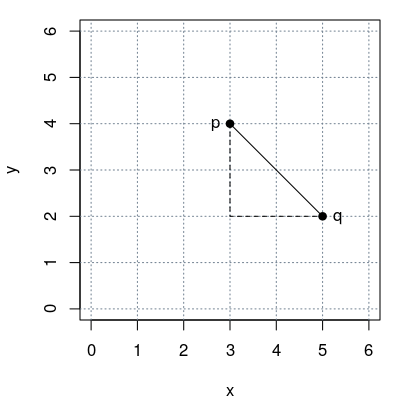## Exam 1

1. #### Question

What is the distance between the two points $p = (3, 4)$ and $q = (5, 2)$ in a Cartesian coordinate system?

#### Solution

The distance $d$ of $p$ and $q$ is given by $d^2 = (p_1 - q_1)^2 + (p_2 - q_2)^2$ (Pythagorean formula).

Hence $d = \sqrt{(p_1 - q_1)^2 + (p_2 - q_2)^2} = \sqrt{(3 - 5)^2 + (4 - 2)^2} = 2.828$.